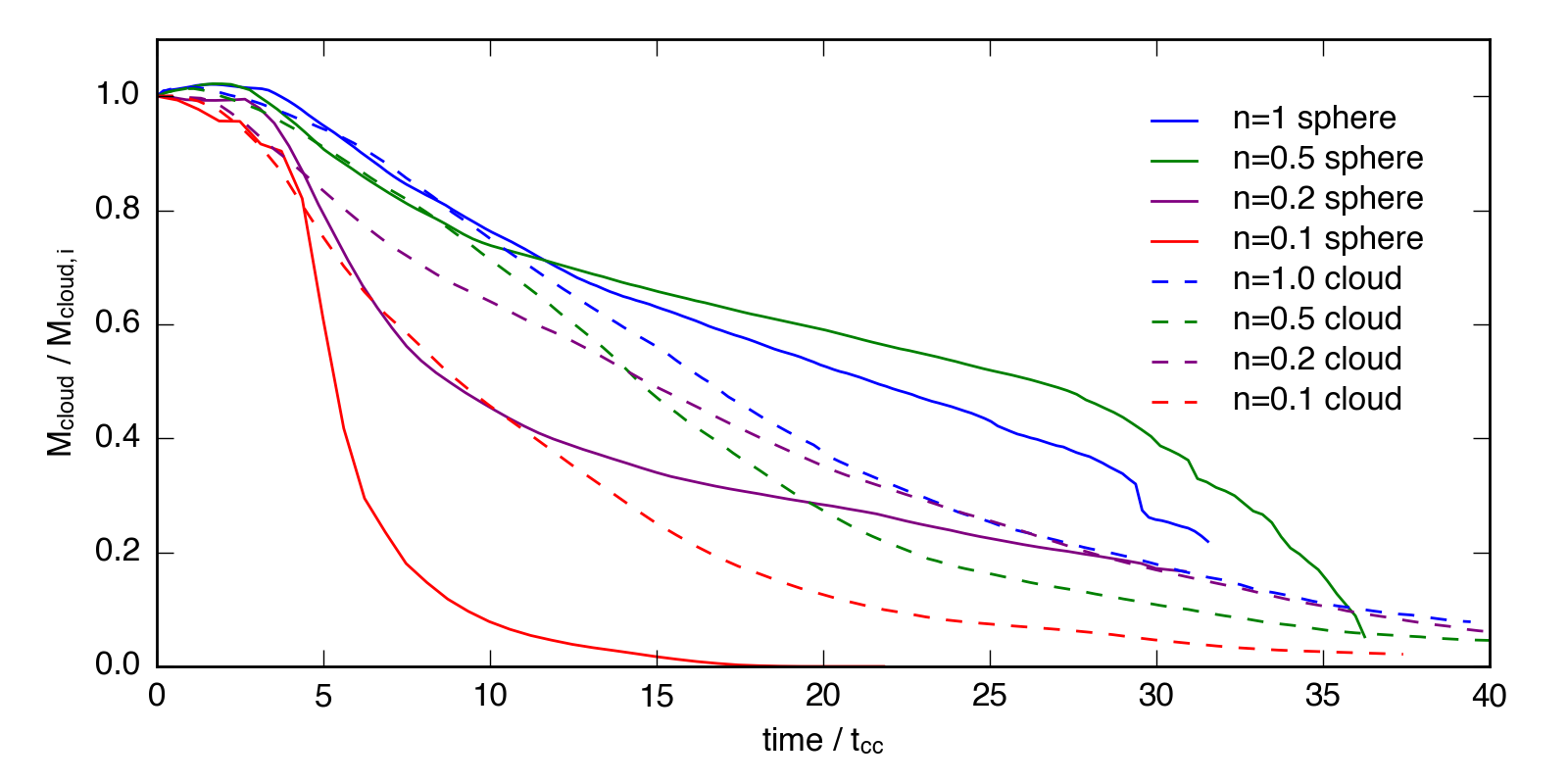# Mass Evolution, Part 3

## May 16, 2016

I’ve now run low resolution (32 cells / $R_\mathrm{cl}$) simulations for all 8 cases: $\tilde{n} = 1$, $\tilde{n} = 0.5$, $\tilde{n} = 0.2$, and $\tilde{n} = 0.1$ for both the sphere and the cloud. The movies can all be found at the appropriate url.

Below is a plot showing the mass evolution of all the low resolution simulations. I’ve used a density threshold of 1/3 $\tilde{n}_\mathrm{i}$ to measure the mass throughout each simulation. In many of these the late-time evolution includes significant high density material leaving the grid, but the early-time evoultion should be relatively complete.I wouldn’t read into this too much, since the mass evolution for the high resolution sims (still running) is different enough that these are clearly not converged. However, I did think it interesting how closely the sphere and cloud evolution track each other for the two high density cases at early times, and that the low-density cloud simulations do not diverge in their evolution as quickly as the clouds (presumably because much of their initial mass is still in material that is high density enough to cool).